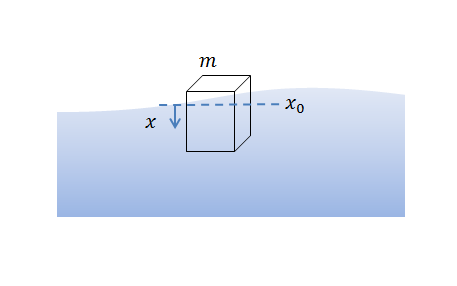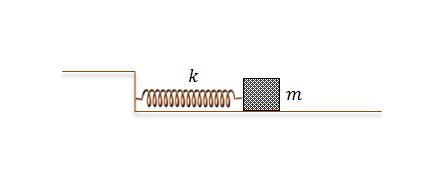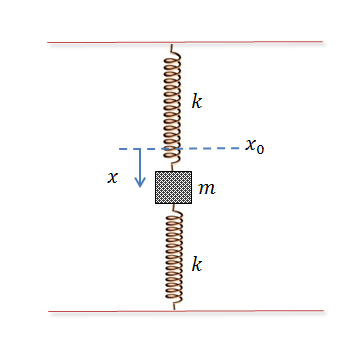Classical Mechanics

# Solutions to Simple Harmonic MotionA $$40\text{ g}$$ cube of edge length $$l=3\text{ cm}$$ floats on water, oscillating up and down. Initially at $$t=0,$$ the position of the body was $$x(t=0) = 5 \text{ cm }$$ and the velocity was $$v(t=0) = 0 \text{ m/s },$$ where $$x$$ denotes the vertical difference in the position of the cube from the equilibrium point $$x_0.$$ What is the velocity $$v(t)$$ of the body at $$t = 2 \text{ s } ?$$

Assumptions and Details

• Positive sign of $$x(t)$$ means downward direction.
• Ignore any friction.
• The density of water is $$\rho = 1 \text{ g/cm}^3.$$
• The gravitational acceleration is $$g=10\text{ m/s}^2.$$As illustrated in the above diagram, a body of mass $$m = 1 \text{ kg}$$ attached to a spring with spring constant $$k = 9 \text{ N/m}$$ is oscillating on a frictionless floor. Initially at $$t=0,$$ the position of the body was $$x(t=0) = 1 \text{ m }$$ and the velocity was $$v(t=0) = 0 \text{ m/s },$$ where $$x$$ denotes the difference in the length of the spring from its original length.

What is the position of the body at $$t = 3 \text{ s } ?$$A $$40\text{ g}$$ cube of edge length $$l=5\text{ cm}$$ floats on water, oscillating up and down. Initially at $$t=0,$$ the position of the body was $$x(t=0) = 4 \text{ cm }$$ and the velocity was $$v(t=0) = 0 \text{ m/s },$$ where $$x$$ denotes the vertical difference in the position of the cube from the equilibrium point $$x_0.$$ What is $$x(t)$$ at $$t = 2 \text{ s } ?$$

Positive sign of $$x(t)$$ means downward direction.
Ignore any friction.
The density of water is $$\rho = 1 \text{ g/cm}^3.$$
The gravitational acceleration is $$g=10\text{ m/s}^2.$$As illustrated in the above diagram, a body of mass $$m = 3 \text{ kg}$$ attached to a spring with spring constant $$k = 75 \text{ N/m}$$ is oscillating on a frictionless floor. Initially at $$t=0,$$ the position of the body was $$x(t=0) = 1 \text{ m }$$ and the velocity was $$v(t=0) = 0 \text{ m/s },$$ where $$x$$ denotes the difference in the length of the spring from its original length. What is the velocity of the body at $$t = 5 \text{ s } ?$$A body of mass $$m = 6 \text{ kg}$$ is oscillating on two identical springs with spring constant $$k = 48 \text{ N/m},$$ as shown in the above diagram, where $$x$$ denotes the downward difference from the equilibrium point $$x_0.$$ Initially, the difference of the body was $$x(t=0) = 4 \text{ m }$$ and the velocity was $$v(t=0) = 0 \text{ m/s }.$$ What is the position of the body at $$t = 4 \text{ s } ?$$

Assumptions and Details

• The gravitational acceleration is $$g = 10 \text{m/s}^2.$$
×